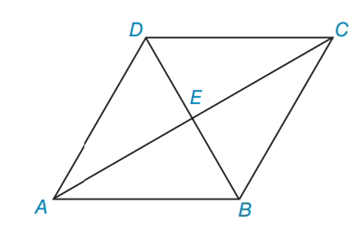Chapter 4.3, Problem 16EElementary Geometry For College St...

7th Edition
Alexander + 2 others
ISBN: 9781337614085

Solutions

Chapter
SectionElementary Geometry For College St...

7th Edition
Alexander + 2 others
ISBN: 9781337614085
Textbook Problem

In Exercises 15 to 18, consider rhombus ABCD with diagonals A C ¯  and  D B ¯ . When the answer is not a whole number, leave a square root answer.Exercises 15 – 18If AE = 6 and EB = 5, find AB.

To determine

To find:

AB of the given rhombus.

Explanation

The basic properties of rhombus:

1) All the properties of a parallelogram apply (the ones that matter here are parallel sides, opposite angles are congruent, and consecutive angles are supplementary).

2) All sides are congruent by definition.

3) The diagonals bisect the angles.

4) The diagonals are perpendicular bisectors of each other.

Calculation:

Given: AE = 6 and EB = 5

Consider the rhombus.

To find AB:

From the property, in rhombus, diagonals are perpendicular bisectors.

In rhombus ABCD, AEB is right triangle.

By using Pythagorean Theorem

Still sussing out bartleby?

Check out a sample textbook solution.

See a sample solution

The Solution to Your Study Problems

Bartleby provides explanations to thousands of textbook problems written by our experts, many with advanced degrees!

Get Started

In Exercises 4562, find the values of x that satisfy the inequality (inequalities). 60. 2x3x14

Applied Calculus for the Managerial, Life, and Social Sciences: A Brief Approach

30. If a variable x is normally distributed, with and

Mathematical Applications for the Management, Life, and Social Sciences

Simplify: 3064

Elementary Technical Mathematics

Solve the equations in Exercises 112 for x (mentally, if possible). ax+b=c(a0)

Finite Mathematics and Applied Calculus (MindTap Course List)

In Exercises 7-12, solve for y in terms of x. (y3)2=5+(x+1)2

Calculus: An Applied Approach (MindTap Course List)

Differentiate. f(x) = (3x2 5x)ex

Single Variable Calculus: Early Transcendentals

The graph of x = cos t, y = sin2 t is:

Study Guide for Stewart's Single Variable Calculus: Early Transcendentals, 8th